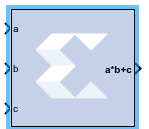## Vivado Design Suite Reference Guide: Model-Based DSP Design Using System Generator (UG958)

Document ID
UG958
Release Date
2020-11-18
Version
2020.2 English

This block is listed in the following Xilinx® Blockset libraries: Floating-Point, Math, and Index.

The Xilinx MultAdd block performs both fixed-point and floating-point multiply and addition with the a and b inputs used for the multiplication and the c input for addition or subtraction.If the MultAdd inputs are floating point, then inputs a,b, and c must be of the same data type. If the inputs are fixed point, then the port c binary point must be aligned to the sum of the port a and port b binary points.

## Block Parameters

The block parameters dialog box can be invoked by double-clicking the icon in your Simulink® model.

Basic tab
Parameters specific to the Basic tab are as follows.
Operation
Specifies that an addition will be performed after multiplication.
Subtraction
Specifies that a subtraction will be performed after multiplication.
Adds a subtract port to the block, which controls whether the operation following multiplication is addition or subtraction (subtract High = subtraction, subtract Low = addition).
Optional Ports
Provide enable port
Adds an active-High enable port to the block interface.
Latency
Latency
This defines the number of sample periods by which the block's output is delayed. The latency values you can set depend on whether you are performing fixed point or floating point arithmetic:
• For fixed point arithmetic, you can only specify a latency of 0 (for no latency) or -1 (for maximum/optimal latency). If you have added an enable port to the block interface, you can only specify a latency of -1 for fixed point arithmetic.
• For floating point arithmetic, you can only specify a latency of 0 (for no latency) or a positive integer. If you have added an enable port to the block interface, you can only specify a positive integer for floating point arithmetic.

See the LogiCORE IP Multiply Adder v3.0 Product Guide for details on latency in the block.

Output tab

Parameters specific to the Output tab are as follows.

Precision
This parameter allows you to specify the output precision for fixed-point arithmetic. Floating point arithmetic output will always be Full precision.
Full
The block uses sufficient precision to represent the result without error.
User Defined
If you do not need full precision, this option allows you to specify a reduced number of total bits and/or fractional bits.
Fixed-point Output Type
Arithmetic type
Signed (2’s comp)
The output is a Signed (2’s complement) number.
Unsigned
The output is an Unsigned number.

Other parameters used by this block are explained in the topic Common Options in Block Parameter Dialog Boxes.